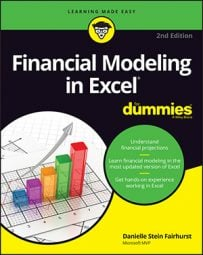##### Financial Modeling in Excel For DummiesToday well over 400 functions are available in Excel, and Microsoft keeps adding more with each new version of the software. Many of these functions aren't relevant for use in finance, and most Excel users only use a very small percentage of the available functions. If you're using Excel for the purpose of financial modeling, you need a firm grasp on the most commonly used functions, at the very least.

Although there are many, many more that you'll find useful when building models, here's a list of the most basic functions that you can't be without.

 Function What It Does SUM Adds up, or sums together, a range of cells. MIN Calculates the minimum value of a range of cells. MAX Calculates the maximum value of a range of cells. AVERAGE Calculates the average value of a range of cells. ROUND Rounds a single number to the nearest specified value, usually to a whole number. ROUNDUP Rounds up a single number to the nearest specified value, usually to a whole number. ROUNDDOWN Rounds down a single number to the nearest specified value, usually to a whole number. IF Returns a specified value only if a single condition has been met. IFS Returns a specified value if complex conditions have been met. COUNTIF Counts the number of values in a range that meet a certain single criterion. COUNTIFS Counts the number of values in a range that meet multiple criteria. SUMIF Sums the values in a range that meet a certain single criterion. SUMIFS Sums the values in a range that meet multiple criteria. VLOOKUP Looks up a range and returns the first corresponding value in a vertical table that matches exactly the specified input. HLOOKUP Looks up a range and returns the first corresponding value in a horizontal table that matches exactly the specified input. An error is returned if it cannot find the exact match. INDEX Works like the coordinates of a map and returns a single value based on the column and row numbers you input into the function fields. MATCH Returns the position of a value in a column or a row. Modelers often combine MATCH with the INDEX function to create a lookup function, which is far more robust and flexible and uses less memory than the VLOOKUP or HLOOKUP. PMT Calculates the total annual payment of a loan. IPMT Calculates the interest component of a loan. PPMT Calculates the principal component of a loan. NPV Takes into account the time value of money by giving the net present value of future cash flows in today's dollars, based on the investment amount and discount rate.

There's a lot more to being a good financial modeler than simply knowing lots of Excel functions. A skilled modeler can select which function is best to use in which situation. Usually, you can find several different ways to achieve the same result, but the best option is always the function or solution that is the simplest, clearest, and easiest for others to understand.